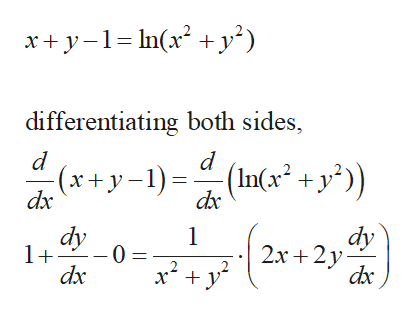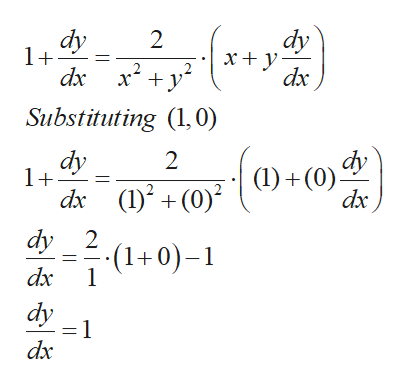Find an equation of the tangent line to the graph of the function at the given point.

Question

Find an equation of the tangent line to the graph of the function at the given point.

Step 1

For the given numerical,

(1) We will calculate the slope at the given point

(2) Use the slope point form with the given coordinates

(3) Derive the final answer.

Step 2

To calculate the slope of the given function, differentiate the same with respect to x.help_outlineImage Transcriptionclosexy-1n2 + y^) differentiating both sides d (In(x2 + y°)) - = (x+y-1) dx dx 1 dy -0 = x2y dx dy 2х + 2у dxc 1+ fullscreen
Step 3

Substitute (1,0) to calculate value of...help_outlineImage Transcriptionclosedy dy 1+ 2 dx Substituting 0) dy 1+ dy 2 dx ()(0) dx ()(0))(0). dy 2 (1+0)- dx 1 dy =1 dx fullscreen

Want to see the full answer?

See Solution

Want to see this answer and more?

Our solutions are written by experts, many with advanced degrees, and available 24/7

See Solution
Tagged in

Functions